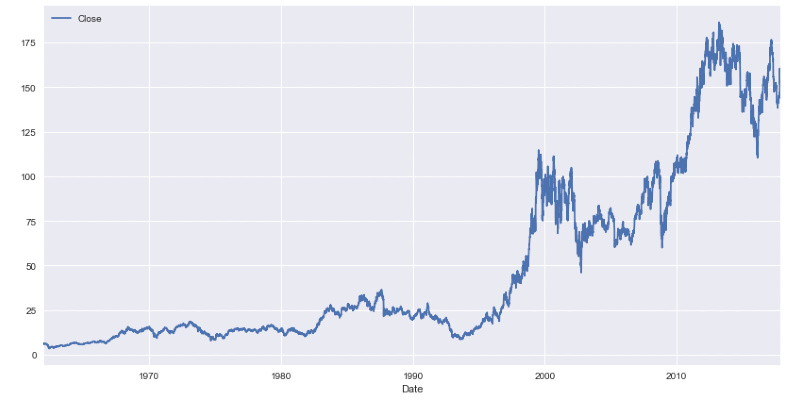# Time Series AnalysisDuración
6-9 horas

Time series are used in a multitude of areas, being increasingly frequent that the generation of data is accompanied by a time stamp. For example, the output of a sensor that measures the temperature of an engine is recorded together with information about the date and time of generation of the data, allowing us to apply specialized techniques that predict engine breakdown or a decrease in performance before it occurs. The applications of this type of analysis are innumerable: from the predictive analysis of the evolution of vital signs of a patient, to the prediction of the evolution of a stock market value.

We will begin this course with an introduction to the most basic techniques on which this type of methodologies are based and we will delve into the ARIMA family of algorithms.

For the correct follow-up of the course attendees must know the Python programming language.

### Contents:

• Introduction to time series analysis
• Basic methods of predicting time series
• Mathematical basis
• Correlation
• Autocorrelation
• Autocorrelation function
• Partial autocorrelation function
• White noise
• Self-regressive models
• AIC and BIC
• Predictions
• AR series profile
• Moving Average Models
• MA series profile
• The ARMA model
• Series stationarity
• Augmented Dicky-Fuller test
• Transformations
• Integration
• The ARIMA model
• Best model searches
• Time series decomposition
• The SARIMA model
• Model diagnosis
• Automation of the calculation of optimal orders
• The SARIMAX model
• Pandas Library:
• Temporal data types
• Temporary data processing tools